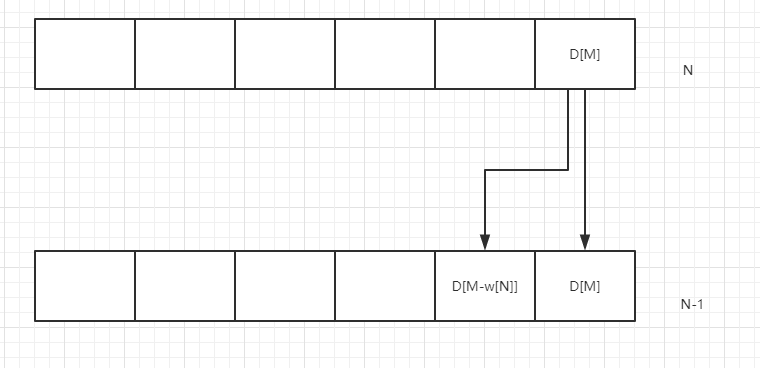# 动态规划之01背包

## 动态规划之01背包

#### 2.分析题意

• 装备一个手镯就会加魅力值和负重
• 要求魅力值最大，负重有一个上限，不同负重可能装备多种不同的手镯
##### 2.1状态

ps.这里的列不是代表单纯只放这种类型手镯，而包括了前面放的手镯。

##### 2.3图表分析

1 4 7 12 16 19 23
2 0 7 12 13 19 19
3 0 7 12 12 19 19
4 0 7 7 7 7 7

D25要看D35和D33，D33+d2=18，D35=19，故D25=19。

##### 2.4简化#### 3.AC代码

#include<iostream>
#include<string>
using namespace std;
int w;
int v;
int f;
int max(int x, int y) {
return x > y ? x : y;
}
int main() {
memset(f, 0, sizeof(f));
memset(w, 0, sizeof(w));
memset(v, 0, sizeof(v));
int N, M;
while (cin >> N >> M) {
for (int i = 1; i <= N; i++) {
cin >> w[i] >> v[i];
}
for (int i = N; i >0; i--) {
for (int j = M; j > 0; j--) {
if (j >= w[i]) {
f[j] = max(f[j - w[i]] + v[i], f[j]);
}
}
}
cout << f[M] << endl;
}
return 0;
}

posted @ 2019-05-14 20:17  WhiteBlackCat  阅读(142)  评论(0编辑  收藏  举报